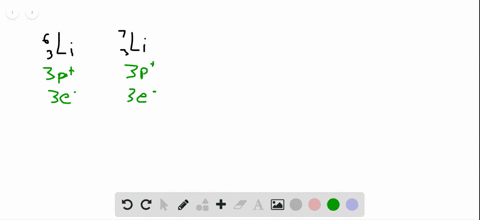Sign up for our free STEM online summer camps starting June 1st!View Summer Courses### The nucleus of 6 Li is a powerful absorber of neu…

03:38Manhattan College

Need more help? Fill out this quick form to get professional live tutoring.

Get live tutoring
Problem 92

Identify the element represented by each of the following symbols and give the number of protons and neutrons in each: $(\mathbf{a})_{33}^{74} X,(\mathbf{b}) \frac{1}{33} X$$(\mathbf{c})_{63}^{152} \mathrm{X},(\mathbf{d}) \frac{209}{83} \mathrm{X}$.

## Discussion

You must be signed in to discuss.

## Video Transcript

guys Region Chapter two Problem 92. Chemistry of central signs. So they want to identify the element represent by each of falling symbols and give member of protons and neutrons and each. So let's hear what the number of protons and neutrons. So as so the protons are always a number on the bottom of the in these kind of scenarios. So we have 33 protons, 53 protons, 63 protons and a three pro tennis now for neutrons. If the's top number, the math number and we subtract that number of protons. So this one is going to be 40 41 neutrons. This one is going to be 74 neutrons, and this one's going to be 89 neutrons, and this one is going to be 126 neutrons. So let's look at which elements that we're dealing with. So which element has 33 protons? That is going to be arsenic. The gentleman has 53 protons That is going to be iodine. Gentlemen, it has 63 new 63 protons that is going to be European, and you and which element has 89 83 protons. That's going to be business